Summary: Equilibrium of Rigid Bodies (Coplanar Concurrent System)

# Summary: Equilibrium of Rigid Bodies (Coplanar Concurrent System) - Notes | Study Engineering Mechanics - Mechanical Engineering

 1 Crore+ students have signed up on EduRev. Have you?

Types of Forces

Forces that act on a body can be divided into two general categories—

• Applied forces (action)
• Reactive forces (or, simply reactions)

Reaction

Reaction is the opposing force that a support offers whenever it is acted upon by external or inherent forces.

Free Body Diagram

Free body diagram is a diagram in which a rigid body is isolated from the system and all active forces applied to the body and reactive forces as a result of mechanical contact are represented.

Examples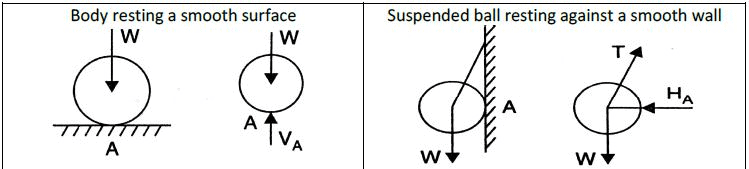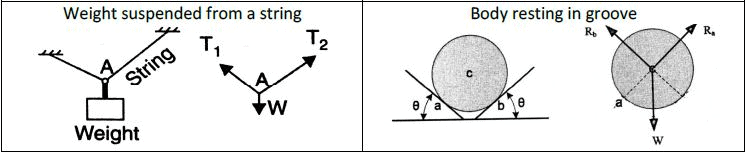Steps for Drawing Free Body Diagram1. A sketch of the body is drawn assuming that all supports (surfaces of contact, supporting cables, etc.) have been removed.2. All applied forces (including weight) and support reactions are drawn and labeled on the sketch.3. Apply the weight of the body to its center of gravity (if it is uniform, then apply it to the centroid). If the sense of a reaction is unknown, it should be assumed

A. EQUILBRIUM OF RIGID BODIES

A rigid body is said to be in equilibrium if the resultant of all external and reactive forces and moments acting on it is zero.

Lami’s Theorem

If three coplanar concurrent forces acting on a body keep it in equilibrium, then each force is proportional to the sine of the angle between the other two”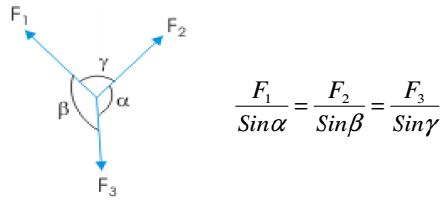Note: Lami’s theorem is applicable only to 3 coplanar concurrent forces in equilibrium

Proof

By applying polygon law of forces, draw triangle OAB representing the system of forces shown with external angles as indicated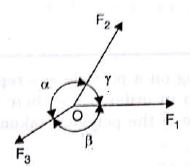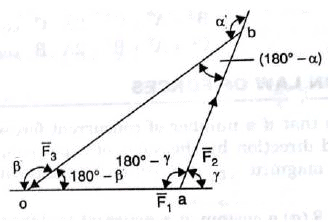Applying Sine Law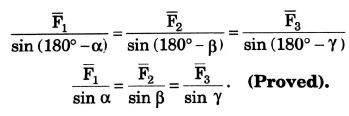General Equations of Equilibrium

1. The algebraic sum of all forces in a force system is zero.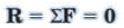2. The algebraic sum of all moments in a force system is zero.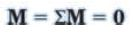Equations of Equilibrium For Coplanar Systems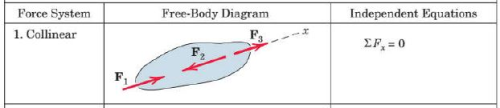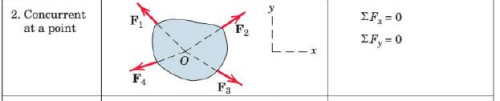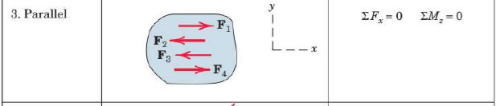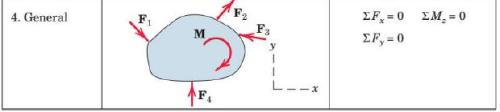Solving Equilibrium Problems1. Draw proper Free- Body Diagram2. Resolve all the forces into x and y components3. Apply Equilibrium conditions along the x and y directions4. Solve the resultant algebraic equationsIn case of moments, try to select the point you take moments around such that the line of action of at least one unknown force passes through that point. This will eliminate one unknown from your moment equation and will result in simpler equations to work with.
The document Summary: Equilibrium of Rigid Bodies (Coplanar Concurrent System) - Notes | Study Engineering Mechanics - Mechanical Engineering is a part of the Mechanical Engineering Course Engineering Mechanics.
All you need of Mechanical Engineering at this link: Mechanical Engineering

## Engineering Mechanics

24 videos|71 docs|58 tests
 Use Code STAYHOME200 and get INR 200 additional OFF

## Engineering Mechanics

24 videos|71 docs|58 tests

Track your progress, build streaks, highlight & save important lessons and more!

,

,

,

,

,

,

,

,

,

,

,

,

,

,

,

,

,

,

,

,

,

;